# Repliscope

Repliscope is an R package for creating, normalising, comparing and plotting DNA replication timing profiles. The analysis pipeline starts with BED-formatted read count files (output of localMapper) obtained by high-throughput sequencing of DNA from replicating and non-replicating cells. There are three methods of measuring DNA replication dynamics using relative copy number (Fig.1): sort-seq, sync-seq and marker frequency analysis (MFA-seq). Sort-seq uses fluorescence-activated cell sorting (FACS) to enrich for non-replicating and replicating cells from an asynchronous population. Sync-seq requires cells to be arrested in non-replicating cell cycle phase (i.e. G1), followed by release into S phase. Samples are then taken throughout S phase when cells synchronously synthesise DNA according to the replication timing programme. In the case of MFA-seq, rapidly dividing cells in exponential growth phase are directly used as the replicating sample, while a saturated culture serves as a non-replicating control sample. While the latter approach of obtaining cells is the simplest, it also requires deeper sequencing due to decreased dynamic range and, thus, is more suitable for organisms with small genomes (typically, bacteria).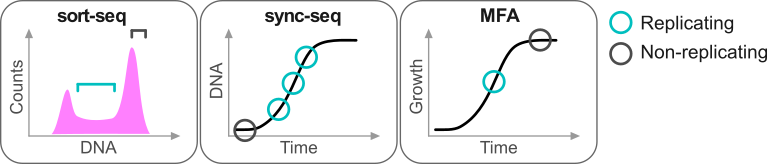## Analysis overview

For best experience, use the Repliscope in interactive mode. To do so, simply run the runGUI() function.

The typical command line analysis using Repliscope starts with loading BED-formatted read count files using the loadBed function. Various functions allow removal of genomic bins containing low quality data (rmChr,rmOutliers). To aid read count analysis, two visualisation functions are used: plotBed and plotCoverage. Next, read-depth adjusted ratio of reads from the replicating sample to reads in the non-replicating sample is calculated using the makeRatio function; the resulting ratio values are distributed around one. The normaliseRatio function is then used to transpose the ratio values to a biologically relevant relative DNA copy number scale (typically, from 1 to 2). The normalised ratio values are, essentially, replication timing profiles, where low values indicate late replication and high values - early replication. The plotRatio function helps to visualise the ratio values as a histogram. Genomic bins containing unusually high or low ratio values may be removed using the trimRatio function. smoothRatio uses cubic spline to smooth replication profile data. compareRatios can be used to calculate difference between two replication profiles using z-score statistics. Finally, replication profiles are plotted using the plotGenome function, which also allows for various genome annotations.

## runGUI()

The runGUI function launches the package in interactive mode as a Shiny app. This is the recommended mode for the majority of applications.

### Usage

runGUI()

### Examples

runGUI()

The loadBed function reads BED-formatted files into an R session. The BED format specifications can be found at UCSC. When read using this function, The localMapper output BED files typically look like this:

chrom chromStart chromEnd name score
chrI 0 1000 W303_G2 481
chrI 1000 2000 W303_G2 311
chrI 2000 3000 W303_G2 471
chrI 3000 4000 W303_G2 1298
chrI 4000 5000 W303_G2 1124

The BED file fields may be separated by tabs or spaces. If the BED file contains a header, it will be ignored. If a genome dataframe is provided, only data for the chromosomes/regions within it will be retained (this may be used to split genomes containing many chromosomes into several plots). The resulting data is ordered by “chromStart” columns, retaining original chromosome order, unless a genome dataframe is provided.

### Usage

loadBed(file,genome,name)
• file Path to the BED file (string, required).
• genome A mask dataframe to exclude data from the BED file (dataframe, optional). The genome dataframe must contain the ‘chrom’ column and may further contain ‘chromStart’ and ‘chromEnd’ columns, in this order.
• name A string to replace the ‘name’ column of the loaded BED file (string, optional).

### Examples

W303_G2 <- loadBed(system.file("extdata/W303_G2.bed",package="Repliscope"), name='W303_G2')

W303_G2_chrI <- loadBed(system.file("extdata/W303_G2.bed",package="Repliscope"), name='W303_G2',genome=sacCer3[["genome"]])

## rmChr()

A function to remove single chromosome data from a bed dataframe

### Usage

rmChr(bed,chr)
• bed A bed dataframe containing ‘chrom’ column (dataframe, required).
• chr Chromosome to remove (string, required).

### Examples

W303_S <- rmChr(W303_S,"chrM")

## rmOutliers()

A function to remove outliers from the “score” column of a supplied bed dataframe. There are three methods available: max, IQR and median. Max is used to remove 1 or more maximum values; IQR uses the InterQuartile Range to detect outliers, while the median method can be used to remove data based on the genome-wide median.

### Usage

rmOutliers(bed,method,n=1,range=3,loLim=0.25,hiLim=NULL)
• bed A dataframe containing the ‘score’ column (dataframe, required).
• method Method to detect outliers: “max”, “IQR” or “median” (string, required).
• n Number of max values to remove (integer, optional, defaults to 1). Use with “max” method.
• range Number of IQR above the 3rd or below the 1st IQR to set the threshold (double, optional, defaults to 3). Use with “IQR” method.
• loLim Low limit for the median method (double, optional, defaults to 0.25).
• hiLim High limit for the median method (double, optional).

### Examples

bedDF <- rmOutliers(W303_S,method="max",n=2)

bedDF <- rmOutliers(W303_S,method="IQR",range=3)

bedDF <- rmOutliers(W303_S,method="median",loLim=0.25,hiLim=2)

## plotBed()

A function to plot values in the ‘score’ column of the supplied bed dataframe, a boxplot per unique chromosome name in the ‘chrom’ column. The resulting plot also highlights outliers based on the interquartile range. The genome wide median is plotted as a pink line through the boxplots.

plotBed(W303_G2)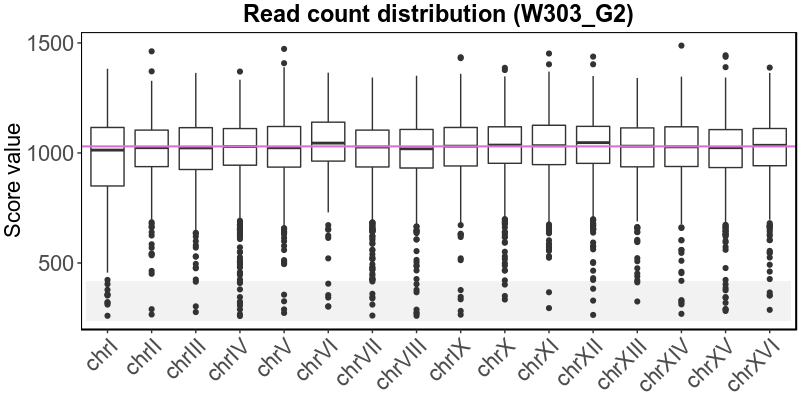### Usage

plotBed(bed,plotting=TRUE)
• bed A dataframe containing ‘score’ and ‘chrom’ columns (dataframe, required).
• plotting Should the plot object be sent to the default device? (boolean, required, defaults to TRUE).

### Examples

plotBed(W303_S)

plotObject <- plotBed(W303_G2,plotting=FALSE)

## plotCoverage()

A function to create a scatterplot of values in the ‘score’ column of the supplied bed dataframe, along chromosome coordinates. The genome wide median is plotted as a pink line.

plotCoverage(W303_S,region='chrII:0-813136')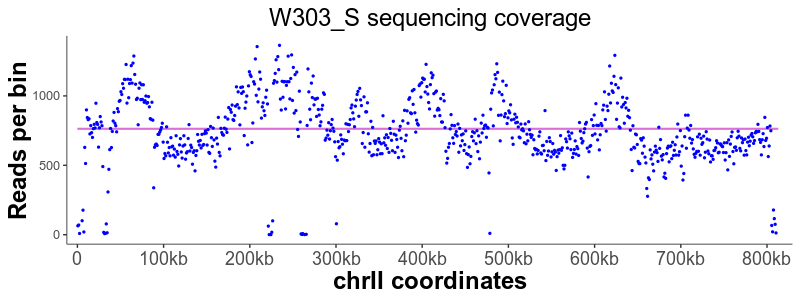### Usage

plotCoverage(bed,region=FALSE,plotting=TRUE)
• bed A dataframe containing ‘score’,‘chrom’,‘chromStart’ and ‘chromEnd’ columns (dataframe, required).
• region Only plot for the provided region in the format ‘chrI:1000-3000’ (string, optional).
• plotting Should the plot object be sent to the default device? (boolean, required, defaults to TRUE).

### Examples

plotCoverage(W303_G2)

plotObject <- plotCoverage(W303_S,plotting=FALSE)

## makeRatio()

A function to calculate the ‘score’ ratio between two bed dataframes. It merges two supplied bed dataframes, calculates the ratios of their “score” values and normalises the ratios by the ‘score’ total sums.

chrom chromStart chromEnd name.rep name.nonRep ratio ratioFactor
chrI 0 1000 W303_S W303_G2 0.7682 1.0
chrI 1000 2000 W303_S W303_G2 0.6678 1.0
chrI 2000 3000 W303_S W303_G2 0.7277 1.0
chrI 3000 4000 W303_S W303_G2 0.7411 1.0
chrI 4000 5000 W303_S W303_G2 0.6994 1.0

### Usage

makeRatio(bedRep,bedNonRep)
• bedRep Bed dataframe containing read counts from a replicating sample (dataframe, required).
• bedNonRep Bed dataframe containing read counts from a non-replicating sample (dataframe, required).

Both bed dataframes must contain “chrom”,“chromStart”, “chromEnd” and “score” columns.

### Examples

ratioDF <- makeRatio(W303_S,W303_G2)

## normaliseRatio()

A function to normalise ratio values to fit a biologically relevant scale. It scales values from the ‘ratio’ column of the provided dataframe either using a supplied ‘rFactor’ value or automatically to best fit the scale from one to two.

### Usage

normaliseRatio(ratioDF,rFactor=NULL,replace=TRUE)
• ratioDF A ratio dataframe containing the ‘ratio’ column (dataframe, required).
• rFactor Value to normalise by, related to replication progression (numeric, optional).
• replace Should the existing ‘ratio’ values be overwritten or stored in a new ‘tmpRatio’ column (boolean, required, defaults to TRUE).

### Examples

ratioDF <- normaliseRatio(W303)
ratioDF <- normaliseRatio(W303,rFactor=1.41,replace=FALSE)

## plotRatio()

A function that plots a histogram of values in a supplied vector using ggplot2 and highlights the interval between 1 and 2 in green.

plotRatio(W303norm$ratio)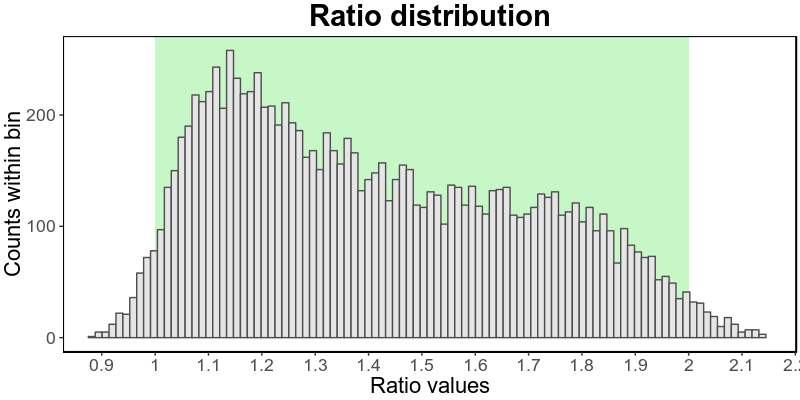### Usage plotRatio(ratio,plotting=TRUE) • ratio A numeric vector containing raw or smoothed ratio values (numeric vector, required). • plotting Should the plot object be sent to the default device? (boolean, required, defaults to TRUE). ### Examples plotRatio(W303$ratio)
plotObject <- plotRatio(W303\$ratio,plotting=FALSE)

## trimRatio()

A function to remove outliers from the “ratio” column of a supplied ratio dataframe.

### Usage

trimRatio(ratioDF,loLim,hiLim)
• ratioDF A ratio dataframe containing the ‘ratio’ column (dataframe, required).
• loLim Low limit threshold (double, required).
• hiLim High limit threshold (double, required).

### Examples

W303 <- trimRatio(W303,0.5,1.5)

## smoothRatio()

A function to smooth ratio values using a cubic smoothing spline. The smoothRatio function splits values from the ‘ratio’ column by chromosome and based the supplied groupMin and split parameters and then applies the smooth.spline function from R stats package. The supplied dataframe may contain multiple ratios, i.e. ratios produced using multiple replicating samples and/or multiple non-replicating samples. This must be reflected in the ‘name.rep’ and ‘name.nonRep’ columns. In other words, different ratio dataframes may be merged using the rbind function before calling the smoothRatio function.

### Usage

smoothRatio(ratioDF,groupMin=5,split=5)
• ratioDF A ratio dataframe or combined ratios dataframe containing the ‘ratio’ column (dataframe, required).
• groupMin Minimum number of values required to make a group (integer, required, defaults to 5).
• split Minimum number of adjacent bins with missing values to close current group (integer, required, defaults to 5).

### Examples

ratioDF <- smoothRatio(W303norm)

ratioDFs <- smoothRatios(rbind(Dbf4myc,W303norm))

## compareRatios()

A function to compare two replication profiles. compareRatios takes two ratio dataframes that were binned the same way and uses z-score statistics to find p-values of their differences. The function outputs a combined dataframe containing the two input ratio dataframes in a long format with an added ‘p.value’ column.

### Usage

compareRatios(ratio1,ratio2)
• ratio1 Ratio dataframe, or a string containing name of a ratio dataframe (dataframe or string, required).
• ratio2 Ratio dataframe, or a string containing name of a ratio dataframe (dataframe or string, required).

### Examples

ratioDFs <- compareRatios(W303norm,Dbf4myc)

## plotGenome

plotGenome plots scatterplot/barplot/polygon of the ‘score’ and/or the ‘splineSmooth’ column values by genomic coordinates, either for the whole genome or a specified region. It also allows annotation with additional BED-formatted data.

plotGenome(sortSeq,region='chrXII:0-410000',lines=sacCer3[["cen"]],circles=sacCer3[["ori"]])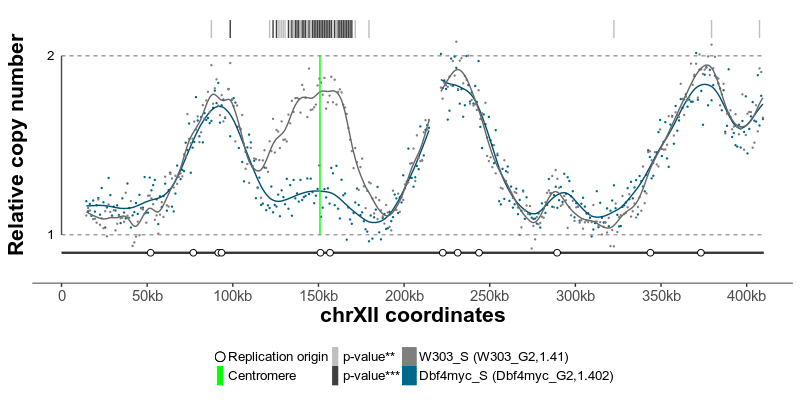### Usage

plotGenome(ratioDFs, geom="geom_point", ylims=c(1,2), plotting=TRUE,
genome=NULL, region=FALSE, guide=NULL,
lines=NULL, circles=NULL, rectangles=NULL, pointers=NULL,
colourLines='#00FF00', colourCircles='#FFFFFF', colourRectangles='#FF0000', colourPointers='#FF7F00'
)
• ratioDFs A ratio dataframe or combined ratios dataframe containing ‘ratio’ column (dataframe, required).
• geom ggplot2 geom to use for plotting: “geom_point”,“geom_ribbon” or “geom_segment” (string, required, defaults to “geom_point”).
• ylims A vector of two values for y axis limits - first is lowest, second is highest (numeric vector, required, defaults to 1 and 2)
• plotting Should the plot object be sent to the default device? (boolean, required, defaults to TRUE).
• genome A mask dataframe to exclude data from the ratio dataframe (dataframe, optional). #’ The genome dataframe must contain “chrom”,“chromStart” and “chromEnd” columns.
• region Only plot the provided region in the format ‘chrI:1000-3000’ (string, optional).
• guide A dataframe guiding the plotGenome function how to plot the data (dataframe, optional). The guide dataframe must contain the following columns: ‘order’ (integer) - order to plot data, ‘name.rep’ (character) - replicating sample name that matches the one in the ratioDFs dataframe, ‘name.nonRep’ (character) - non-replicating sample name that matches the one in the ratioDFs dataframe, ‘raw’ (logical) - should the raw raw data be plotted, ‘smooth’ (logical) - should the smoothed data be plotted, ‘color’(character) - R colour to plot the current sample, both raw and smoothed data.
• lines Additionally plot vertical lines from a BED formatted dataframe (dataframe, optional).
• circles Additionally plot circles on the chromosome line from a BED formatted dataframe (dataframe, optional).
• rectangles Additionally plot rectangles on the chromosome line from a BED formatted dataframe (dataframe, optional).
• pointers Additionally plot downward pointing triangles from a BED formatted dataframe (dataframe, optional).
• colourLines Colour for ‘lines’ data (string, required, defaults to green).
• colourCircles Colour for ‘circles’ data (string, required, defaults to white).
• colourRectangles Colour for ‘rectangles’ data (string, required, defaults to red).
• colourPointers Colour for ‘pointers’ data (string, required, defaults to orange).

### Examples

plotGenome(sortSeq,genome=sacCer3[["genome"]],lines=sacCer3[["cen"]],circles=sacCer3[["ori"]])

plotGenome(sortSeq, geom="geom_ribbon", guide=guide,region="chrIX:250000-439885", lines=sacCer3[["cen"]], circles=sacCer3[["ori"]])

plotGenome(syncSeq[["data"]], geom="geom_segment", guide=syncSeq[["guide"]], genome=sacCer3[["genome"]], lines=sacCer3[["cen"]], circles=sacCer3[["ori"]], colourLines="black")